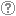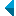# Search the repositoryQuery: search in TitleAuthorAbstractKeywordsFull textYear of publishing ANDORAND NOT search in TitleAuthorAbstractKeywordsFull textYear of publishing ANDORAND NOT search in TitleAuthorAbstractKeywordsFull textYear of publishing ANDORAND NOT search in TitleAuthorAbstractKeywordsFull textYear of publishing Work type: All work types Habilitation (m4) Specialist thesis (m3) High school thesis (m6) Bachelor work * (dip) Master disertations * (mag) Doctorate disertations * (dok) Research Data or Corpuses (data) * old and bologna study programme Language: All languagesSlovenianEnglishGermanCroatianSerbianBosnianBulgarianCzechFinnishFrenchGerman (Austria)HungarianItalianJapaneseLithuanianNorwegianPolishRussianSerbian (cyrillic)SlovakSpanishSwedishTurkishUnknown Search in: RUP    FAMNIT - Faculty of Mathematics, Science and Information Technologies    FHŠ - Faculty of Humanities    FM - Faculty of Management    FTŠ Turistica - Turistica – College of Tourism Portorož    FVZ - Faculty of Health Sciences    IAM - Andrej Marušič Institute    PEF - Faculty of Education    UPR - University of Primorska    ZUP - University of Primorska PressCOBISS    Fakulteta za humanistične študije, Koper    Fakulteta za management Koper in Pedagoška fakulteta Koper    Fakulteta za vede o zdravju, Izola    Knjižnica za tehniko, medicino in naravoslovje, Koper    Turistica, Portorož    Znanstveno-raziskovalno središče Koper Options: Show only hits with full text Reset

 1 - 10 / 6512345671.On distance-regular Cayley graphs for abelian groupsŠtefko Miklavič, 2012, invited lecture at foreign universityFound in: osebiPublished: 15.10.2013; Views: 2131; Downloads: 76Full text (0,00 KB) 2.The Terwilliger algebra of a distance-regular graph of negative typeŠtefko Miklavič, 2009, original scientific articleAbstract: Let ▫$\Gamma$▫ denote a distance-regular graph with diameter ▫$D \ge 3$▫. Assume ▫$\Gamma$▫ has classical parameters ▫$(D,b,\alpha,\beta)▫$ with ▫$b < -1$▫. Let ▫$X$▫ denote the vertex set of ▫$\Gamma$▫ and let ▫$A \in {\mathrm{Mat}}_X(\mathbb{C})$▫ denote the adjacency matrix of ▫$\Gamma$▫. Fix ▫$x \in X$▫ and let $A^\ast \in {\mathrm{Mat}}_X(\mathbb{C})$ denote the corresponding dual adjacency matrix. Let ▫$T$▫ denote the subalgebra of ${\mathrm{Mat}}_X(\mathbb{C})$ generated by ▫$A,A^\ast$▫. We call ▫$T$▫ the Terwilliger algebra of ▫$\Gamma$▫ with respect to ▫$x$▫. We show that up to isomorphism there exist exactly two irreducible ▫$T$▫-modules with endpoint 1; their dimensions are ▫$D$▫ and ▫$2D-2$▫. For these ▫$T$▫-modules we display a basis consisting of eigenvectors for ▫$A^\ast$▫, and for each basis we give the action of ▫$A$▫.Found in: osebiKeywords: distance-regular graph, negative type, Terwilliger algebraPublished: 15.10.2013; Views: 2728; Downloads: 105Full text (0,00 KB) 3.On bipartite Q-polynomial distance-regular graphs with c [sub] 2 [equal] 1Štefko Miklavič, 2007, original scientific articleAbstract: Let ▫$\Gamma$▫ denote a bipartite ▫$Q$▫-polynomial distance-regular graph with diameter ▫$d \ge 3$▫, valency ▫$k \ge 3$▫ and intersection number ▫$c_2=1$▫. We show that ▫$\Gamma$▫ has a certain equitable partition of its vertex set which involves ▫$4d-4$▫ cells. We use this partition to show that the intersection numbers of ▫$\Gamma$▫ satisfy the following divisibility conditions: (I) ▫$c_{i+1}-1$▫ divides ▫$c_i(c_i-1)$▫ for ▫$2 \le i \le d-1$▫, and (II) ▫$b_{i-1}-1$▫ divides ▫$b_i(b_i-1)$▫ for ▫$1 \le i \le d-1$▫. Using these divisibility conditions we show that ▫$\Gamma$▫ does not exist if ▫$d=4$▫.Found in: osebiKeywords: mathematics, grah theory, distance-regular graphs, ▫$Q$▫-polynomial property, equitable partitionsPublished: 15.10.2013; Views: 3246; Downloads: 35Full text (0,00 KB) 4.Consistent cyclesŠtefko Miklavič, 2005, invited lecture at foreign universityFound in: osebiPublished: 15.10.2013; Views: 1768; Downloads: 29Full text (0,00 KB) 5.Leonard triples and hypercubesŠtefko Miklavič, 2006, invited lecture at foreign universityFound in: osebiPublished: 15.10.2013; Views: 1833; Downloads: 59Full text (0,00 KB) 6.The A-like matrices for a HypercubesŠtefko Miklavič, Paul Terwilliger, 2011, original scientific articleFound in: osebiKeywords: A-like matrices, distance-regular graphs, HypercubesPublished: 15.10.2013; Views: 2741; Downloads: 64Full text (0,00 KB)This document has more files! More... 7.Q-polynomial distance-regular graphs with a [sub] 1 [equal] 0 and a [sub] 2 [not equal] 0Štefko Miklavič, 2008, original scientific articleAbstract: Let ▫$\Gamma$▫ denote a ▫$Q$▫-polynomial distance-regular graph with diameter ▫$D \ge 3$▫ and intersection numbers ▫$a_1=0$▫, ▫$a_2 \ne 0$▫. Let ▫$X$▫ denote the vertex set of ▫$\Gamma$▫ and let ▫$A \in {\mathrm{Mat}}_X ({\mathbb{C}})$▫ denote the adjacency matrix of ▫$\Gamma$▫. Fix ▫$x \in X$▫ and let denote $A^\ast \in {\mathrm{Mat}}_X ({\mathbb{C}})$ the corresponding dual adjacency matrix. Let ▫$T$▫ denote the subalgebra of ▫$A{\mathrm{Mat}}_X ({\mathbb{C}})$▫ generated by ▫$A$▫, ▫$A^\ast$▫. We call ▫$T$▫ the Terwilliger algebra of ▫$\Gamma$▫ with respect to ▫$x$▫. We show that up to isomorphism there exists a unique irreducible ▫$T$▫-module ▫$W$▫ with endpoint 1. We show that ▫$W$▫ has dimension ▫$2D-2$▫. We display a basis for ▫$W$▫ which consists of eigenvectors for ▫$A^\ast$▫. We display the action of ▫$A$▫ on this basis. We show that ▫$W$▫ appears in the standard module of ▫$\Gamma$▫ with multiplicity ▫$k-1$▫, where ▫$k$▫ is the valency of ▫$\Gamma$▫.Found in: osebiKeywords: mathematics, graph theory, adjacency matrix, distance-regular graph, Terwilliger algebraPublished: 15.10.2013; Views: 3242; Downloads: 25Full text (0,00 KB) 8.Consistent Cycles in 1/2-Arc-Transitive GraphsŠtefko Miklavič, Marko Boben, Primož Potočnik, 2009, original scientific articleFound in: osebiKeywords: mathematics, graph theory, 1/2-arc-transitivity, consistent cyclePublished: 15.10.2013; Views: 3494; Downloads: 35Full text (0,00 KB)This document has more files! More... 9.Leonard triples and hypercubesŠtefko Miklavič, 2007, original scientific articleAbstract: Let ▫$V$▫ denote a vector space over ▫$\mathbb{C}$▫ with finite positive dimension. By a Leonard triple on ▫$V$▫ we mean an ordered triple of linear operators on ▫$V$▫ such that for each of these operators there exists a basis of ▫$V$▫ with respect to which the matrix representing that operator is diagonal and the matrices representing the other two operators are irreducible tridiagonal. Let ▫$D$▫ denote a positive integer and let ▫${\mathcal{Q}}_D$▫ denote the graph of the ▫$D$▫-dimensional hypercube. Let ▫$X$ denote the vertex set of ▫${\mathcal{Q}}_D$▫ and let ▫$A \in {\mathrm{Mat}}_X ({\mathbb{C}})$▫ denote the adjacency matrix of ▫${\mathcal{Q}}_D$▫. Fix ▫$x \in X$▫ and let ▫$A^\ast \in {\mathrm{Mat}}_X({\mathbb{C}})$▫ denote the corresponding dual adjacency matrix. Let ▫$T$▫ denote the subalgebra of ▫${\mathrm{Mat}}_X({\mathbb{C}})$ generated by ▫$A,A^\ast$▫. We refer to ▫$T$▫ as the Terwilliger algebra of ▫${\mathcal{Q}}_D$▫ with respect to ▫$x$▫. The matrices ▫$A$▫ and ▫$A^\ast$▫ are related by the fact that ▫$2iA = A^\ast A^\varepsilon - A^\varepsilon A^\ast$▫ and ▫$2iA^\ast = A^\varepsilon A - AA^\varepsilon$▫, where ▫$2iA^\varepsilon = AA^\ast - A^\ast A$▫ and ▫$i^2 = -1$▫. We show that the triple ▫$A$▫, ▫$A^\ast$▫, ▫$A^\varepsilon$▫ acts on each irreducible ▫$T$▫-module as a Leonard triple. We give a detailed description of these Leonard triples.Found in: osebiKeywords: mathematics, graph theory, Leonard triple, distance-regular graph, hypercube, Terwilliger algebraPublished: 15.10.2013; Views: 2914; Downloads: 115Full text (0,00 KB) 10.Classification of tetravalent distance magic circulant graphsŠtefko Miklavič, Primož Šparl, 2021, original scientific articleFound in: osebiKeywords: distance magic labeling, distance magic graph, circulant graphPublished: 18.10.2021; Views: 283; Downloads: 19Full text (0,00 KB)
Search done in 0 sec.